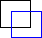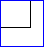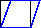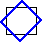# Affine Transformation

## Linear mapping method using affine transformation

Affine transformation is a linear mapping method that preserves points, straight lines, and planes. Sets of parallel lines remain parallel after an affine transformation.

The affine transformation technique is typically used to correct for geometric distortions or deformations that occur with non-ideal camera angles. For example, satellite imagery uses affine transformations to correct for wide angle lens distortion, panorama stitching, and image registration. Transforming and fusing the images to a large, flat coordinate system is desirable to eliminate distortion. This enables easier interactions and calculations that don’t require accounting for image distortion.

The following table illustrates the different affine transformations: translation, scale, shear, and rotation.

Affine Transform Example Transformation Matrix

Translation$\left[\begin{array}{c}1 & 0 & 0\\0 & 1 & 0\\ t_x & t_y & 1\end{array}\right]$

$$t_x$$ specifies the displacement along the $$x$$ axis

$$t_y$$ specifies the displacement along the $$y$$ axis.

Scale$\left[\begin{array}{c}s_x & 0 & 0\\0 & s_y & 0\\ 0 & 0 & 1\end{array}\right]$

$$s_x$$ specifies the scale factor along the $$x$$ axis

$$s_y$$ specifies the scale factor along the $$y$$ axis.

Shear$\left[\begin{array}{c}1 & sh_y & 0\\sh_x & 1 & 0\\ 0 & 0 & 1\end{array}\right]$

$$sh_x$$ specifies the shear factor along the $$x$$ axis

$$sh_y$$ specifies the shear factor along the $$y$$ axis.

Rotation$\left[\begin{array}{c}\cos(q) & \sin(q) & 0\\-\sin(q) & \cos(q) & 0\\ 0 & 0 & 1\end{array}\right]$

$$q$$ specifies the angle of rotation.

 $$t_x$$

Table referenced from “Using a Transformation Matrix” section of 2D Geometric Transformations.

For more details about affine transformation, see the Image Processing Toolbox™ documentation.

See also: image analysis, color profile, image thresholding, image enhancement, image reconstruction, image segmentation, image transform, image registration, digital image processing, image processing and computer vision, Steve on Image Processing, lab color, point cloud, 3D Image Processing